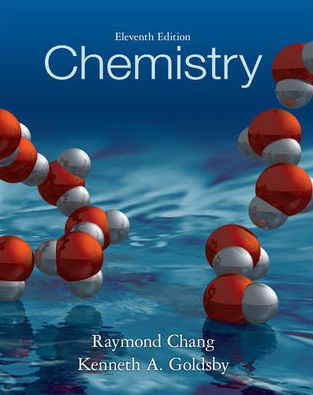×
×

# Solutions for Chapter 20: Chemistry 11th Edition## Full solutions for Chemistry | 11th Edition

ISBN: 9780073402680Solutions for Chapter 20

Solutions for Chapter 20
4 5 0 357 Reviews
12
2
##### ISBN: 9780073402680

Chemistry was written by and is associated to the ISBN: 9780073402680. Since 94 problems in chapter 20 have been answered, more than 318379 students have viewed full step-by-step solutions from this chapter. Chapter 20 includes 94 full step-by-step solutions. This textbook survival guide was created for the textbook: Chemistry, edition: 11. This expansive textbook survival guide covers the following chapters and their solutions.

Key Chemistry Terms and definitions covered in this textbook
• alpha (a) helix

For proteins, a feature of secondary structure that forms when a portion of the protein twists into a spiral.

• amine

Compounds containing a nitrogen atom that is connected to one, two, or three alkyl or aryl groups.

• amines.

Organic bases that have the functional group —NR2, where R may be H, an alkyl group, or an aromatic group. (24.4)

• chemical equilibrium.

A state in which the rates of the forward and reverse reactions are equal. (14.1)

• Cycloalkane

A saturated hydrocarbon that contains carbons joined to form a ring

• Deshielding

The term used to express the concept of less shielding in NMR

• flagpole interactions

For cyclohexane, the steric interactions that occur between the flagpole hydrogen atoms in a boat conformation.

• functional group

A characteristic group of atoms/bonds that possess a predictable chemical behavior.

• pascal (Pa)

The SI unit of pressure: 1 Pa = 1 N >m2 . (Section 10.2)

• physical changes

Changes (such as a phase change) that occur with no change in chemical composition. (Section 1.3)

• pi (p) bond

A bond formed from adjacent, overlapping p orbitals.

• quartet

In NMR spectroscopy, a signal that is comprised of four peaks.

• reactant

A starting substance in a chemical reaction; it appears to the left of the arrow in a chemical equation. (Section 3.1)

• Reaction coordinate diagram

A graph showing the energy changes that occur during a chemical reaction; energy is plotted on the vertical axis and reaction progress is plotted on the horizontal axis.

• Resonance

A theory that many molecules and ions are best described as a hybrid of several Lewis structures

• resonance hybrid

A term used to describe the character of a chemical entity (molecule, ion, or radical) exhibiting more than one significant resonance structure.

• Signal

A recording in an NMR spectrum of a nuclear magnetic resonance

• standard atomic weight

The weighted averages for each element, which takes into account isotopic abundance.

• Sulfi de

The sulfur analog of an ether; a molecule containing a sulfur atom bonded to two carbon atoms. Sulfi des are also called thioethers

• trivalent

An element, such as nitrogen, that forms three bonds.

×